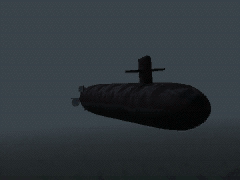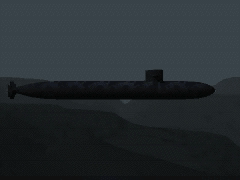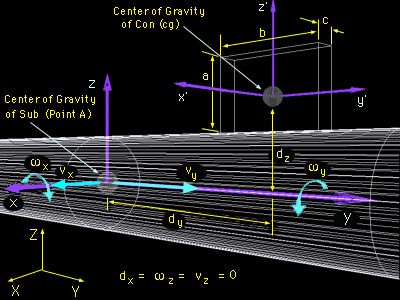Ch 9. 3-D Motion of a Rigid Body Multimedia Engineering Dynamics Translating Coordinates Rotating Coordinates Inertia & Ang. Momentum Equationsof Motion
 Chapter - Particle - 1. General Motion 2. Force & Accel. 3. Energy 4. Momentum - Rigid Body - 5. General Motion 6. Force & Accel. 7. Energy 8. Momentum 9. 3-D Motion 10. Vibrations Appendix Basic Math Units Basic Equations Sections Search eBooks Dynamics Fluids Math Mechanics Statics Thermodynamics Author(s): Kurt Gramoll ©Kurt GramollDYNAMICS - CASE STUDY IntroductionProblem Graphic - Skew ViewProblem Graphic - Side View The designers of an attack sub are predicting its performance during dive maneuvers. When designing the coning tower, they must determine its contribution to the angular momentum about the center of gravity of the sub. What is known: At the instant the dive commences, the components of the velocity and angular velocity of the sub relative to the earth are      vXI, vYJ, wYJ, -wXK (vZK = wZK = 0). The dimensions of the sub and the coordinate system for the sub and the earth are shown in the diagram. Question At the instant the dive commences, what are the inertial properties of the coning tower about the center of gravity of the sub? At that same instant, what is the angular momentum of the coning tower about the center of gravity of the sub?Problem Diagram Approach Model the con as a homogeneous rectangular prism. Determine the inertial properties of the con about its center of gravity, then transfer them to the center of gravity of the sub. Use the inertial properties of the con about the center of gravity of the sub to determine the angular momentum of the con about the center of gravity of the sub.

Practice Homework and Test problems now available in the 'Eng Dynamics' mobile app
Includes over 400 problems with complete detailed solutions.
Available now at the Google Play Store and Apple App Store.